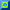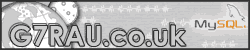HOMEMY SHACKSOFTWARECLX BASICSM5BSZCALCSLINKSMAPS-ESMAPS-ALLUSER AREAG7RAU - CALCSCALCULATORS Below are a selection of calculators for use in ham radioMUF CALCULATOR An assistance tool for calculating the probability of es happening on 2mQRA CALCULATOR Calculating QRA to latitude / longitude and visa-versaQRB CALCULATOR Calculate distance between 2 locatorsWATTS TO DB Calculating watts to decibels and visa-versa